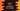# How to find the exponential value for a number in Python## How to find the exponential value for a number in Python:

In this post, we will learn how to find the exponential value for a number in Python. The exponential value for a number is calculated by multiplying the same number for a given number of times.

The number is called base number and the number of times it is multiplied to itself is called exponent.

For example, if the base is 3 and exponent is 4, the exponential value will be 3 * 3 * 3 * 3.

We can find the exponential value in different ways in Python. Let’s have a look at them one by one:

## Method 1: By using exponential operator ** :

The exponential operator ** can be used to find the exponential value. We have to use it as like base ** exponent.

Let me show you an example of this operator:

``````base = 2
exponent = 4

result = base ** exponent

print(f'Base: {base}, Exponent: {exponent}, Result: {result}')``````

In this example, we are finding the exponential of a given base and exponent value. If you run this program, it will print the below output:

``Base: 2, Exponent: 4, Result: 16``

### Example with user given values:

We can also take the values as inputs from the user to calculate the exponential:

``````base = int(input('Enter the value of base: '))
exponent = int(input('Enter the value of exponent: '))

result = base ** exponent

print(f'Base: {base}, Exponent: {exponent}, Result: {result}')``````

If you run this program, it will take the base and exponent as inputs from the user and it will print the final result.

``````Enter the value of base: 3
Enter the value of exponent: 4
Base: 3, Exponent: 4, Result: 81``````

### Example with floating point values:

The exponential operator also works with floating point values. For example:

``````base = float(input('Enter the value of base: '))
exponent = float(input('Enter the value of exponent: '))

result = base ** exponent

print(f'Base: {base}, Exponent: {exponent}, Result: {result}')``````

I have changed the above program to take floating point numbers as inputs from the user. It will give output as like below:

``````Enter the value of base: 1.23
Enter the value of exponent: 2.45
Base: 1.23, Exponent: 2.45, Result: 1.6606095188286067``````

## Method 2: By using pow() function:

Python pow() function can be used to find the exponential of a number. It is defined as:

``pow(n, e, m)``
• n is the number or base value.
• e is the exponent value.
• m is a optional number. It is used to calculate the modulus.

We don’t have to calculate the modulus, so we can simply ignore m.

Let’s take a look at the example below:

``````base = int(input('Enter the value of base: '))
exponent = int(input('Enter the value of exponent: '))

result = pow(base, exponent)

print(f'Base: {base}, Exponent: {exponent}, Result: {result}')``````

It is taking the base and exponent values as inputs from the user and finds the exponential by using pow.

If you run this program, it will print output as like below:

``````Enter the value of base: 3
Enter the value of exponent: 4
Base: 3, Exponent: 4, Result: 81``````

It also works with float values:

``````base = float(input('Enter the value of base: '))
exponent = float(input('Enter the value of exponent: '))

result = pow(base, exponent)

print(f'Base: {base}, Exponent: {exponent}, Result: {result}')``````

It will give output as like below:

``````Enter the value of base: 1.23
Enter the value of exponent: 2.45
Base: 1.23, Exponent: 2.45, Result: 1.6606095188286067  ``````

## Method 3: By using math.pow():

math.pow() method is similar to pow(). But it takes only two arguments: the base and exponent value. It is defined as like below:

``pow(b, e)``

Where, b is the base and e is the exponent value. Unlike pow(), math.pow() converts both base and exponent values to float and it returns a float value.

``````import math

base = float(input('Enter the value of base: '))
exponent = float(input('Enter the value of exponent: '))

result = math.pow(base, exponent)

print(f'Base: {base}, Exponent: {exponent}, Result: {result}')``````

It will give similar result.

``````Enter the value of base: 1.23
Enter the value of exponent: 2.45
Base: 1.23, Exponent: 2.45, Result: 1.6606095188286067``````

Now, let me change it to take integer values:

``````import math

base = int(input('Enter the value of base: '))
exponent = int(input('Enter the value of exponent: '))

result = math.pow(base, exponent)

print(f'Base: {base}, Exponent: {exponent}, Result: {result}')``````

We are passing integer values as base and exponent. But the return value of math.pow will be a float:

``````Enter the value of base: 3
Enter the value of exponent: 4
Base: 3, Exponent: 4, Result: 81.0``````

## Method 4: exp() method:

exp method is used to find the exponential of a number with base e. So, for a number n, it will give e^n.

This method is defined in the math module and we have to import math module to use it. For example:

``````import math

print(f'math.exp(2): {math.exp(2)}')
print(f'math.exp(2.3): {math.exp(2.3)}')
print(f'math.exp(-4.3): {math.exp(-4.3)}')``````

It will print:

``````math.exp(2): 7.38905609893065
math.exp(2.3): 9.974182454814718
math.exp(-4.3): 0.013568559012200934``````